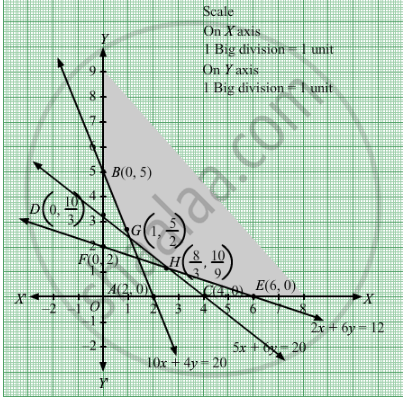# To Maintain One'S Health, a Person Must Fulfil Certain Minimum Daily Requirements for the Following Three Nutrients: Calcium, Protein and Calories. - Mathematics

Sum

To maintain one's health, a person must fulfil certain minimum daily requirements for the following three nutrients: calcium, protein and calories. The diet consists of only items I and II whose prices and nutrient contents are shown below:

 Food I Food II Minimum daily requirement CalciumProteinCalories 1052 466 202012 Price Rs 0.60 per unit Rs 1.00 per unit

Find the combination of food items so that the cost may be minimum.

#### Solution

Let the person takes x units and y units of food I and II respectively that were taken in the diet.
Since, per unit of food I costs Rs 0.60 and that of food II costs Rs 1.00.
Therefore, x lbs of food I costs Rs 0.60x and y lbs of food II costs Rs 1.00y.
Total cost per day = Rs (0.60+1.00y)
​Let Z denote the total cost per day
Then, Z = 0.60+1.00y
Since, each unit of food I contains 10 units of calcium.Therefore, x units of food I contains 10x units of calcium.
Each unit of food II contains 4 units of calcium.So, y units of food II contains 4y units of calcium.
Thus, x units of food I and y units of food II contains (10x + 4y) units of calcium.
But, the minimum requirement is 20 units of calcium.

∴ $10x + 4y \geq 20$

Since, each unit of food I contains 5 units of protein.Therefore, x units of food I contains 5x units of protein.
Each unit of food II contains 6 units of protein.So,y units of food II contains 6y units of protein.
Thus, x units of food I and y units of food II contains (5x + 6y) units of protein.
But, the minimum requirement is 20 lbs of protein.

∴  $5x + 6y \geq 20$

Since, each unit of food I contains 2 units of calories.Therefore, x units of food I contains 2x units of calories.
Each unit of food II contains 6 units of calories.So,y units of food II contains 6y units of calories.
Thus, x units of food I and y units of food II contains (2x + 6y) units of calories.
But, the minimum requirement is 12 lbs of calories.$\therefore 2x + 6y \geq 12$

Finally, the quantities of food I and food II are non negative values.
So,

$x, y \geq 0$

Hence, the required LPP is as follows:
Min Z = 0.60x + 1.00y
subject to

$10x + 4y \geq 20$
$5x + 6y \geq 20$
$2x + 6y \geq 12$
$x, y \geq 0$

First, we will convert the given inequations into equations, we obtain the following equations:
10x + 4y = 20, 5x +6y = 20, 2x + 6y =12, x = 0 and y = 0
Region represented by 10x + 4y ≥ 20:
The line 10x + 4y = 20 meets the coordinate axes at A(2, 0) and B(0, 5) respectively. By joining these points we obtain the line
10x + 4y = 20.Clearly (0,0) does not satisfies the inequation 10x + 4y ≥ 20. So,the region in xy plane which does not contain the origin represents the solution set of the inequation 10x + 4y ≥ 20.
Region represented by 5x +6y ≥ 20:
The line 5x +6y = 20 meets the coordinate axes at

$C\left( 4, 0 \right)$ and  $D\left( 0, \frac{10}{3} \right)$ respectively. By joining these points we obtain the line
5x +6y = 20.Clearly (0,0) does not satisfies the 5x +6y ≥ 20. So,the region which does not contains the origin represents the solution set of the inequation 5x +6y ≥ 20.

Region represented by 2x + 6y ≥ 12:
The line 2x + 6y =12 meets the coordinate axes at $E\left( 6, 0 \right)$ and $E\left( 6, 0 \right)$  respectively. By joining these points we obtain the line
2x + 6y =12.Clearly (0,0) does not satisfies the inequation 2x + 6y ≥ 12. So,the region which does not contains the origin represents the solution set of the inequation 2x + 6y≥ 12.
Region represented by x ≥ 0 and y ≥ 0:
Since, every point in the first quadrant satisfies these inequations. So, the first quadrant is the region represented by the inequations x ≥ 0, and ≥ 0.
The feasible region determined by the system of constraints 10x + 4y ≥ 20, 5x +6y ≥ 20, 2x + 6y ≥ 12, x ≥ 0, and y ≥ 0 are as follows.The set of all feasible solutions of the above LPP is represented by the feasible region shaded in the graph.
The corner points of the feasible region are B(0, 5), $\left( 1, \frac{5}{2} \right)$,H $\left( \frac{8}{3}, \frac{10}{9} \right)$ and E $\left( 6, 0 \right)$

The value of the objective function at these points are given by the following table

 Points Value of Z B $0 . 6\left( 0 \right) + 5 = 5$ G $0 . 6\left( 1 \right) + \frac{5}{2} = 3 . 1$ H $0 . 6\left( \frac{8}{3} \right) + \left( \frac{10}{9} \right) = 1 . 6 + 1 . 1 = 2 . 7$ E $0 . 6\left( 6 \right) + \left( 0 \right) = 3 . 6$

We see that the minimum cost is 2.7 which is at $\left( \frac{8}{3}, \frac{10}{9} \right)$.Thus, at minimum cost, $\frac{8}{3}$ units of food I and $\frac{10}{9}$  units of food II should be included in the diet.

Concept: Graphical Method of Solving Linear Programming Problems
Is there an error in this question or solution?

#### APPEARS IN

RD Sharma Class 12 Maths
Chapter 30 Linear programming
Exercise 30.3 | Q 3 | Page 38